# Lauder: Counting Solutions to Equations in Many Variables over Finite Fields

We present an algorithm which allows us to count solutions to a homogeneous equation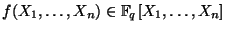of degree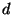(for simplicity we assume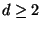,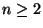) with running time which does not increase exponentially in number of variables. In other words, we are interested in computing the number of projective solutions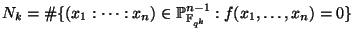for every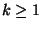. We encode these numbers in the generating function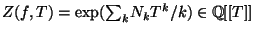which, by a theorem of Dwork, is in fact a rational function. We assume that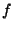is nonsingular, i.e.and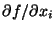for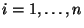have no common projective solution. In this situation, we know that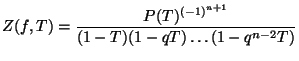where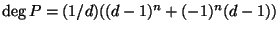.

If we compute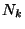naively for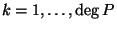then we can of course recover the polynomial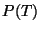; the time required to do this, however, requires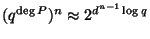evaluations of. The input is given by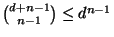terms of size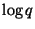, and the output size is approximately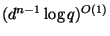. We would like the running time to be polynomial in this quantity.

If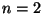, we are counting solutions of a univariate polynomial, and this can be done in time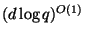. For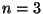, we have an algorithm of Schoof-Pila for curves which has run time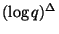where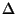depends onexponentially. In general, there is an algorithm (due to L. and Wan) which runs in time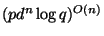. Notice the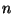in the exponent--we would like to lose this dependence.

The new result: Ifis `sufficiently generic' (we exclude a Zariski closed set which is efficiently computable),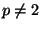, and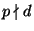, then we can findusing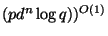bit operations. As a corollary, we see that if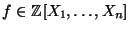is sufficiently generic, then there exists an algorithm which takes as input a prime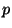, outputs the number of solutions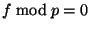, and has run time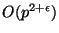.

Recall that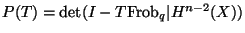, where we write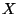for the projective variety defined by the equation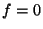. The action of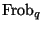can be represented by a matrix with entries in a field of characteristic zero, and we find that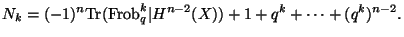For curves, for example, the dimension is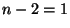, and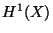is a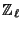-module, for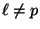.

Instead, we work with the-adic theory, where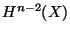is a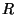-module for a ring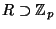. We compute instead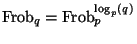, and compute the matrix of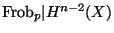. (Specifically,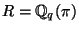where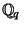is the unramified extension of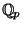of degree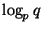and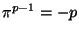. Also ouris actually the primitive part of the cohomology space.) Consider the family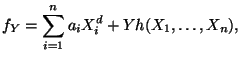and assume that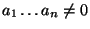. Then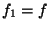and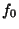is a diagonal form, for which it is easy to count the number of solutions. Now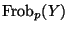is a-adic analytic function with the property thatevaluated at a Teichmuller lift of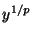is exactly theassociated to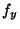.

We see that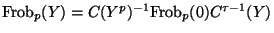where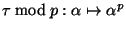, and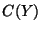is a matrix of power series around the origin satisfying the differential equation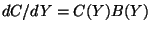with initial condition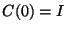, where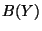is easily computed. This gives a way to computein a radius around the origin, and we need to extend this to the closed disc of radius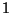.

The entries of the matrices are-adic holomorphic functions, so to compute these modulo a power ofwe find rational functions with denominators corresponding to the values of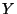where the variety becomes singular and then recover the numerator from the power series. Using overconvergence we get a bound on the degree of these rational functions. We evaluate the rational functions at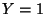. One gets nice complexity because we work with univariate power series, with decay in the coefficients on the order of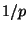, and one needs to take the power ofapproximately on the order of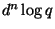.

In the old algorithm, we would work in, the ring of power series in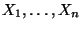over an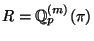where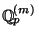is the unramified extension ofof degree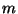(if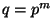) andmodulo an infinite subspace; the power series we must work with have on the order of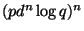terms.

Problem. Find an algorithm which counts the number of points on a curve in time.

Back to the main index for Future directions in algorithmic number theory.